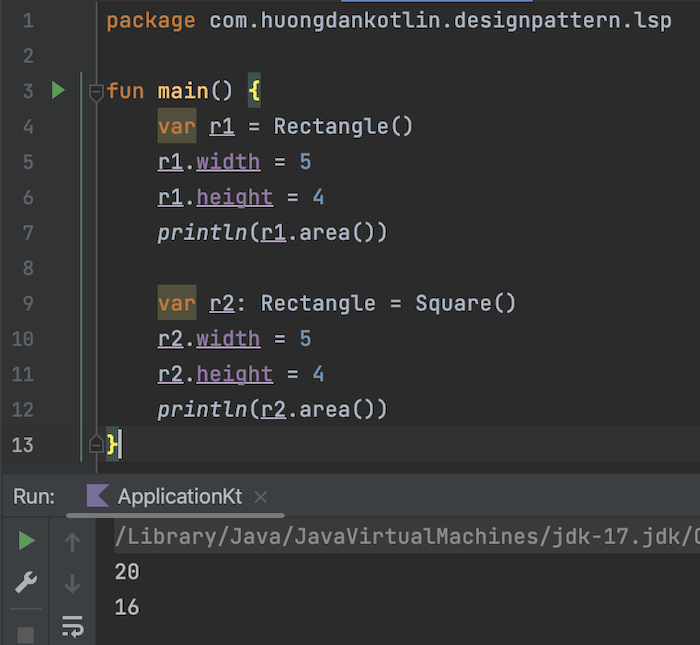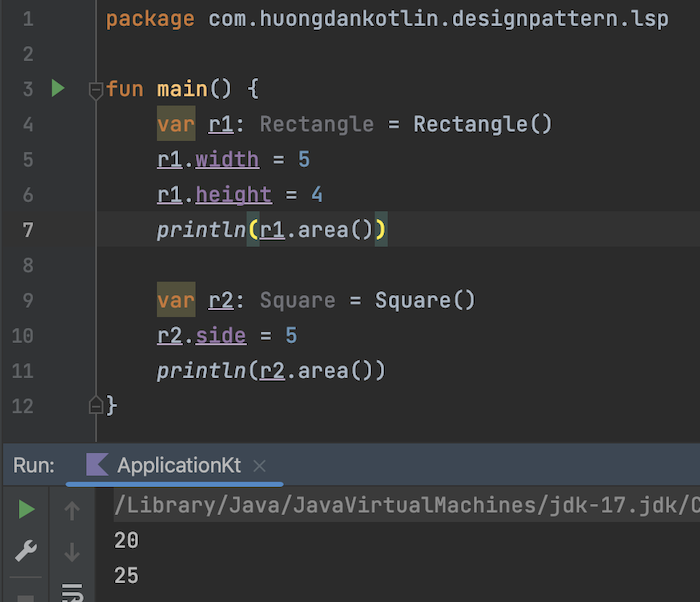# Liskov Substitution Principle in Design Pattern

Liskov Substitution Principle is the principle that when we extend a class, we must ensure the correctness of that class.

An example that you often see when learning about this principle is that a rectangle must be a rectangle, the width and length must always be different:

You should not extend the class that defines a rectangle to build a square class and then define the two sides of the square to be equal:

So that when we instantiate 2 Rectangle objects with 2 different implementations, the results when calculating the area are different, even though they are both rectangles and have the same length and width:

Result:To solve the problem in this case, you should introduce a Shape interface with an abstract method area():

The two classes Rectangle and Square will now implement the Shape interface:

and:

And when calculating the area of the two shapes above:

the result will always be correct: